# Problems of the right triangle

#### Number of problems found: 1297

• ABS trianglePoint S is the center of the square ABCD. The ABS triangle has a content of 9 cm2. What is the circumference of the square in cm?
• Isosceles triangle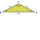Calculate the height of the isosceles triangle ABC with the base AB, AB = c = 10 cm and the arms a = b = 13 cm long.
• Two forces 3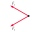Two forces of magnitude 8 Newtons and 15 Newtons respectively act at a point. If the resultant force is 17 Newtons, find the angle between the forces.
• Trapezoid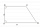The rectangular trapezoid ABCD with right angle at the vertex A has sides a, b, c, d. Calculate the circumference and the area of the trapezoid if given: a = 25cm, c = 10cm, d = 8cm
• Calculate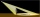Calculate the height of a tree that casts a shadow 22 m long, if you know that at the same time a pillar 2 m high casts a shadow 3 meters long.
• Two angles 2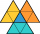Two angles of a triangle have the same measure and the third one is 45 degrees greater than the measure of each of the other two. Find the measure of the largest angle in the triangle.
• Sides in ratio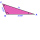The sides of the triangle are in a ratio of 2: 8: 5. Find the dimensions of the remaining sides if the longest side is 32 cm.
• Similarity of triangles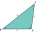If triangle ABC ~ to triangle XYZ, AC = 24, AB = 15, BC = 17, and XY = 9, what is the perimeter of triangle XYZ? Round all sides to 1 decimal place.
• The block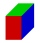The block has the dimensions of 5 cm, 10 cm, and 15 cm. Calculate the size of the wall diagonals of this block.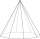You need to make a pad in the shape of a regular octagon with a side length of 4 cm. What is the minimum diameter of the circle-shaped semi-finished product from which we make the pad, and what will be the percentage of waste? (Round the results to 1 deci
• In the 18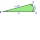In the right triangle ABC, The hypotenuse AB = 15 cm, and B = 25 degree. How long is BC to the nearest centimetre?
• Area of a triangle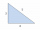Find the area of a triangle with a base of 7 mm and a height of 10 mm?
• Angle ASB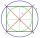On a circle with a radius of 10 cm and with a center S, the points A, B, C are given so that the central angle ASB is 60 degrees and the central angle ASC is 90 degrees. Find the length of the circle arc and the amount of AB and AC offsets.
• Circle inscribed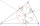There is a triangle ABC and a circle inscribed in this triangle with radius 15. The point T is the point of contact of the inscribed circle with the side BC. What is the area of the triangle ABC if | BT | = 25 a | TC | = 26?
• Bridge diagonally across the river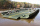The width of the river is 89 m. For terrain reasons, the bridge deviates from a common perpendicular to both banks by an angle of 12° 30 '. Calculate how many meters the bridge is longer than the river.
• Touch circle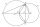Point A has a distance IA, kl = 10 cm from a circle k with radius r = 4 cm and center S. Calculate: a) the distance of point A from the point of contact T if the tangent to the circle is drawn from point A b) the distance of the contact point T from the l
• The perimeterThe perimeter of the triangle is 24, the sides are integers and form an arithmetic sequence. Specify the side sizes of this triangle.
• Approximation of tangent fx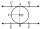What is the non-trig formula (not a polynomial fit) for the growth curve that solves algebraically for the increase between tan(1 degree), tan( 2 degrees) continuing up to tangent(45 degrees)? okay to use pi Check calculation for 12°
• TrapezoidsIn the isosceles trapezoid ABCD we know: AB||CD, |CD| = c = 8 cm, height h = 7 cm, |∠CAB| = 35°. Find the area of the trapezoid.
• Diagonals of rhombus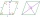Find a length of the diagonal AC of the rhombus ABCD if its perimeter P = 112 dm and the second diagonal BD has a length of 36 dm.

Do you have an exciting math question or word problem that you can't solve? Ask a question or post a math problem, and we can try to solve it.

We will send a solution to your e-mail address. Solved examples are also published here. Please enter the e-mail correctly and check whether you don't have a full mailbox.